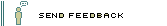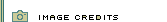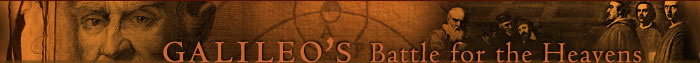His Experiments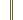Galileo homepage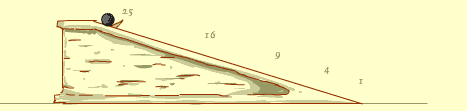Determine the Rate of Acceleration

Using a water clock and an inclined plane, Galileo was able to determine the rate of acceleration due to gravity. With an apparatus like the one above, you could do the same. By timing how long it takes for the ball to roll from the marked distances, you might find out the following: That it takes one unit of time for the ball to roll one unit of distance, two units of time to roll four units of distance, three units of time for the ball to roll nine units of distance, four units of time for the ball to roll 16 units of distance, and five units of time for the ball to roll 25 units of distance.

Which of the following best describes what these measurements reveal?

Galileo's water clock worked like a stopwatch. To start the clock, he allowed water to flow into a container. To stop the clock, he stopped the flow of water. To reset the clock he emptied the container. By weighing the amounts of water in the container, he could then compare the times it took the ball to travel each distance. For example, if twice as much water (in weight) filled the container, he knew that the time measured was twice as long.